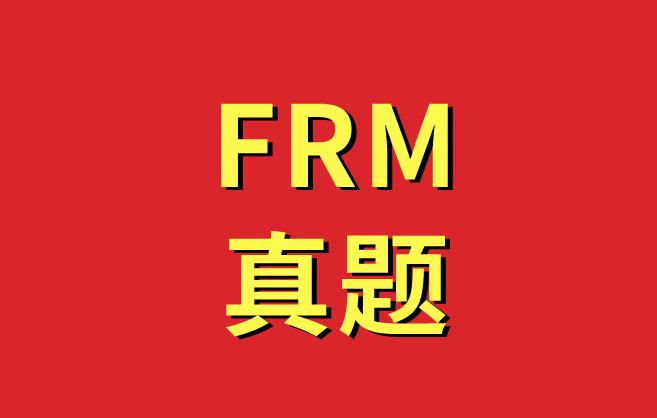2021年新版FRM备考资料下载
• 考纲对比
• 学习计划
• 思维导图
• 复习资料
• 历年真题
• 词典及公式The minimum amount of money that one could expect to lose with a given

probability over a specific period of time is the definition of:

A) Value at risk (VAR).

B) Delta.

C) The hedge ratio.

D) The coefficient of variation.

Aportfolio has a current value of \$40 million and its geometric returns are normally distributed with annual mean of 12.0% and annual volatility (standard deviation) of 40%. Because the geometric returns are normal, the future value of the portfolio has a lognormal distribution. If the returns are i.i.d., what is the 95% confident level, 10-day lognormal value at risk?

A) \$2.91 million

B) \$3.86 million【资料下载】FRM一级思维导图PDF版

C) \$4.77 million

D) \$5.07 million

lognormal VaR = 40 * (1 - exp(0.48% - 8% * 1.645)) =4.76